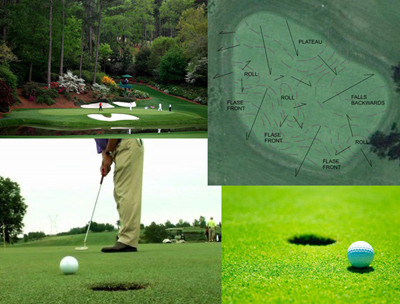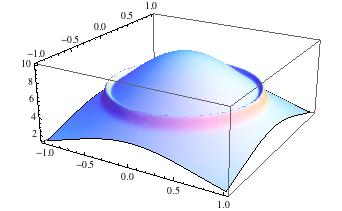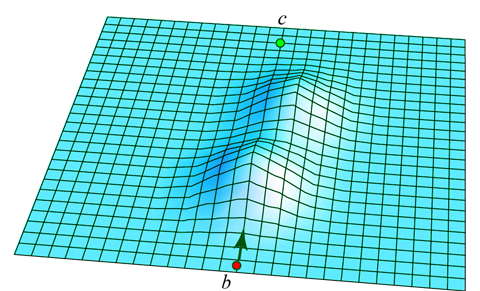# “Rolling Geodesics”: Designing a $k$-putt green

I am interested in what might be called rolling geodesics, paths of physical particles confined to a surface in $\mathbb{R}^3$ under certain force conditions. Here I will pose a specific (but imprecise) recreational question that addresses some aspects these curves: Design a wickedly difficult golf green.Let $D \subset \mathbb{R}^2$ be a unit-radius disk in the plane. Let $h(x,y)$ be a continuous, smooth function defined over $D$; $h(x,y)$ represents the height of a golf green surface $S$ over $(x,y) \in D$. At a point $c \in S$ is centered a golf hole/cup of diameter $\delta$ larger than the diameter of a golf ball, which is sitting at some spot $b \in S$ on the green. The hole should project strictly interior to $D$, as should $b$. The challenge is to design the shape of the green's surface $S=h(x,y)$ to make it extremely difficult to hole a putt.

A putt imparts an instantaneous velocity $v_0$ to the ball at $b$, with the goal that the ball rolls under the influence of gravity and rolling friction with the grass so that its point of contact ends up suspended over the cup hole. Assume that, regardless of its velocity $v(t)$ at that moment, the ball is "sunk," i.e., it falls into the cup.

Some additional assumptions:

• The surface gradient is bounded, e.g., $|\nabla h| \le 1$. (Perhaps 1 is too generous. Rarely is a green sloped more than $10^\circ$.)
• Friction suffices to hold a carefully placed ball stationary-stable at any point $b \in S$.
• The initial velocity $v_0$ is bounded by the requirement that the ball never lifts-off the surface airborn at any point. (See the earlier MO question, Gently falling functions.) This rolling-constraint might be difficult to accommodate, in which case an upperbound $v_{\max}$ could be assumed.
• Assume that $D$'s fringe is surrounded by such a deep drop-off that a ball that leaves the domain of the green can never return.

Here are two specific questions:

Q1. Is there a surface $S$ with cup/pin placement $c \in S$ such that, for some starting point $b \in S$, it is impossible to 1-putt from $b$?

If the answer to Q1 is Yes, then:

Q2. Given any integer $k>1$, is there an $S$ and $c \in S$ that requires $\ge k$ putts to sink the ball from some $b \in S$ into the cup?

Insights under any assumptions (either more or less realistic) welcomed!

(Credit to Alejandro López-Ortiz, who posed several geometric golf questions [but not this specific one] at a conference in August 2011.)

Addendum. Here is Jaap Eldering's example:## 3 Answers

Your constraint on the velocity that the ball may not loose contact with the surface leads to impossible putts: take a vulcano shaped green with the hole at the very top and put a small rim at the foot around the vulcano, for example, take a radially symmetric $h(r) = 10 \exp(-r^2) + \exp(-1000(r-0.8)^2)$. Place the ball (just) outside the rim. A minimum speed to putt is given by potential height energy. On the other hand, if the top of the rim is sharp enough (high second derivative of $h$), then the ball will lift off from the surface there. You could add a constraint on second derivatives of $h$ to prevent such problems.

• @Jaap: Clever! The gradient $\nabla h$ may be too large, but perhaps appropriate scaling would lead to the same impossible puts. Thanks for the example! – – Joseph O'Rourke Dec 22 '11 at 1:03

Some (heuristic) speculation, separate from my previous answer. I'm ignoring the velocity/loss of contact constraint and consider the ball as a point particle, ignoring angular momentum etc. as well.

I think that under these conditions it is always possible to make a 1-putt. Let me assume a sufficiently high initial speed $v_0$ such that the ball never falls still. The problem can then be viewed as a geodesic flow on $T^1 D$. At each point $x \in D \cong S$, the speed is determined by energy conservation, while the (unit) direction of the velocity is specified by $v \in T_x^1 D$. The metric on $T^1 D$ will be dermined by Newton's laws and the height function $h$.

Now let $b$ be the pin position and consider the geodesic flow on $T^1_b D$: the set of all possible directions in which we can shoot the ball. This is topologically a circle and will stay a circle under the continuous flow (assuming that $h$ is sufficiently smooth). Now my speculation is that this circle can be viewed as a "wavefront" that will keep expanding away from $b$ (since the velocity is high enough), and that it will have fully crossed the boundary of $D$ at some time. By continuity, each point on the green then must have been on (I think precisely one) geodesic curve.

• @Jaap: Where are you using the circularity of $\partial D$? Because without that, I believe it is false. Imagine $D$ were a long, thin rectangle, and $S$ is sloped across the width. Then with the cup $c$ near the boundary on the high side, paths of a ball from far away will roll off the low side before reaching $c$. – Joseph O'Rourke Dec 22 '11 at 12:48
• @Joseph: you're right, but I guess that your counterexample only works because the rectangle is not strictly convex. If not both the pin and the cup are at the high side, then by giving the ball a sufficiently high speed, the gravity influence becomes negligable and the ball trajectory will approximate a straight line. This does not work if both pin and cup are located exactly at the high side border, because there must always be some minimal curvature. Note that my argument really is heuristic, I do not directly see whether it can be made more precise. – Jaap Eldering Dec 22 '11 at 14:59

I wonder if surface like this could thwart a 1-putt, with an appropriate velocity limit $v_0 \le v_{\max}$?The cup $c$ is directly ahead of the ball at $b$, directly along the line indicated by the arrow. Clearly putting straight or left of that arrow sends the ball off to the left. It seems that reasonable velocity conditions might ensure that angles $\epsilon$ right of the arrow would still roll left of $c$ from the influence of the left slope of the second knoll, and that aiming further rightward would roll off the right slope of that second knoll.

• I think this does not provide a counterexample provided $h$ is sufficiently smooth. The following argument is also the idea behind my second answer. If you assume that the velocity is always high enough that the ball crosses both hills, then by continuity of the flow, if it can pass $c$ both on the left and right side, by the intermediate value theorem, there must be a solution that reaches $c$. – Jaap Eldering Dec 23 '11 at 19:34
• @Jaap: I see that you are correct, with the key condition that the velocity suffices to reach the peaks of both hills. A lower velocity would, I believe, leave $c$ inaccessible from $b$. Thanks for this insight! – Joseph O'Rourke Dec 24 '11 at 1:05